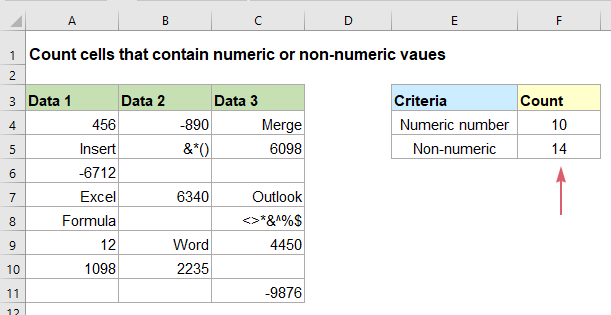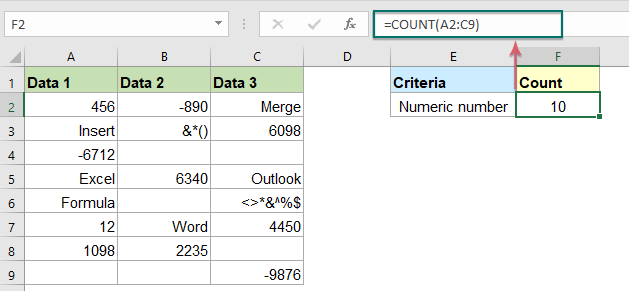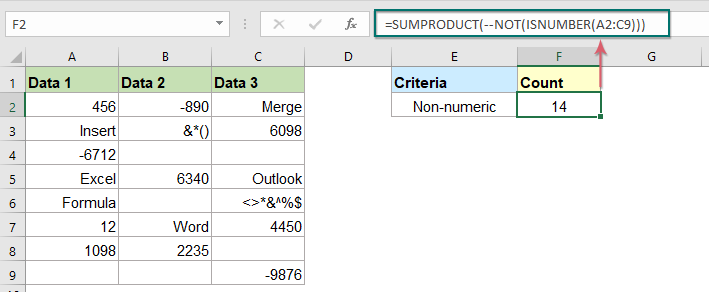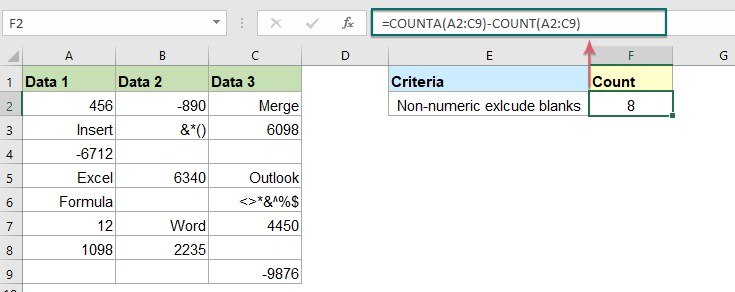Note: The other languages of the website are Google-translated. Back to English

## Count number of cells contain numeric or non-numeric values in Excel

If you have a range of data which contains both numeric and non- numeric values, and now, you may want to count the number of numeric or non-numeric cells as below screenshot shown. This article, I will talk about some formulas for solving this task in Excel.#### Count number of cells contain numeric values

In Excel, the COUNT function can help you to count the number of cells that contain numeric values only, the generic syntax is:

=COUNT(range)
• range: The range of cells that you want to count.

Enter or copy the below formula into a blank cell, and then press Enter key to get the number of numeric values as below screenshot shown:

=COUNT(A2:C9)#### Count number of cells contain non-numeric values

If you want to get the number of cells that contain the non-numeric values, the SUMPRODUCT, NOT and ISNUMBER functions together can solve this task, the generic syntax is:

=SUMPRODUCT(--NOT(ISNUMBER(range)))
• range: The range of cells that you want to count.

Please enter or copy the following formula into a blank cell, and then press Enter key, and you will get the total number of cells with non-numeric values and blank cells, see screenshot:

=SUMPRODUCT(--NOT(ISNUMBER(A2:C9)))##### Explanation of the formula:
• ISNUMBER(A2:C9): This ISNUMBER function searches numbers in the range A2:C9, and returns TRUE or FALSE. So, you will get an array as this:
{TRUE,TRUE,FALSE;FALSE,FALSE,TRUE;TRUE,FALSE,FALSE;FALSE,TRUE,FALSE;FALSE,FALSE,FALSE;TRUE,FALSE,TRUE;TRUE,TRUE,FALSE;FALSE,FALSE,TRUE}.
• NOT(ISNUMBER(A2:C9)): This NOT function converts the above array result in reverse. And the result will like this:
{FALSE,FALSE,TRUE;TRUE,TRUE,FALSE;FALSE,TRUE,TRUE;TRUE,FALSE,TRUE;TRUE,TRUE,TRUE;FALSE,TRUE,FALSE;FALSE,FALSE,TRUE;TRUE,TRUE,FALSE}.
• --NOT(ISNUMBER(A2:C9)): This double negative operator-- converts the above TURE to 1, and FALSE to 0 in the array, and you will get this result: {0,0,1;1,1,0;0,1,1;1,0,1;1,1,1;0,1,0;0,0,1;1,1,0}.
• SUMPRODUCT(--NOT(ISNUMBER(A2:C9)))= SUMPRODUCT({0,0,1;1,1,0;0,1,1;1,0,1;1,1,1;0,1,0;0,0,1;1,1,0}): At last, the SUMPRODUCT function adds all numbers in the array and return the final result: 14.

Tips: With the above formula, you will see all the blank cells will be counted as well, if you just want to get the non-numeric cells without blanks, the below formula can help you:#### Relative function used:

• COUNT:
• The COUNT function is used to count the number of cells that contain numbers, or count the numbers in a list of arguments.
• SUMPRODUCT:
• The SUMPRODUCT function can be used to multiply two or more columns or arrays together, and then get the sum of products.
• NOT:
• The NOT function Returns a reversed logical value.
• ISNUMBER:
• The ISNUMBER function returns TRUE when a cell contains a number, and FALSE if not.

#### More articles:

• Count Number Of Cells That Contain Specific Text In Excel
• Supposing, you have a list of text strings, and may want to find the number of cells that contain certain text as part of their contents. In this case, you can use the wildcard characters (*) that represents any texts or characters in your criteria when applying the COUNTIF function. This article, I will introduce how to use formulas for dealing with this job in Excel.
• Count number of cells not equal to many values in Excel
• In Excel, you may easily get the number of cells not equal to a specific value by using the COUNTIF function, but have you ever tried to count the cells which are not equal to many values? For example, I want to get the total number of the products in column A but exclude the specific items in C4:C6 as below screenshot shown. This article, I will introduce some formulas for solving this job in Excel.
• Count Number Of Cells That Contain Odd Or Even Numbers
• As we all known, odd numbers have a remainder of 1 when divided by 2, and the even numbers have a remainder of 0 when divided by 2. This tutorial, I will talk about how to get the number of cells that contain odd or even numbers in Excel.

### The Best Office Productivity Tools

#### Kutools for Excel - Helps You To Stand Out From Crowd

Would you like to complete your daily work quickly and perfectly? Kutools for Excel brings 300 powerful advanced features (Combine workbooks, sum by color, split cell contents, convert date, and so on...) and save 80% time for you.

• Designed for 1500 work scenarios, helps you solve 80% Excel problems.
• Reduce thousands of keyboard and mouse clicks every day, relieve your tired eyes and hands.
• Become an Excel expert in 3 minutes. No longer need to remember any painful formulas and VBA codes.
• 30-day unlimited free trial. 60-day money back guarantee. Free upgrade and support for 2 years.#### Office Tab - Enable Tabbed Reading and Editing in Microsoft Office (include Excel)

• One second to switch between dozens of open documents!
• Reduce hundreds of mouse clicks for you every day, say goodbye to mouse hand.
• Increases your productivity by 50% when viewing and editing multiple documents.
• Brings Efficient Tabs to Office (include Excel), Just Like Chrome, Firefox, And New Internet Explorer.